# Differentiating Math Instruction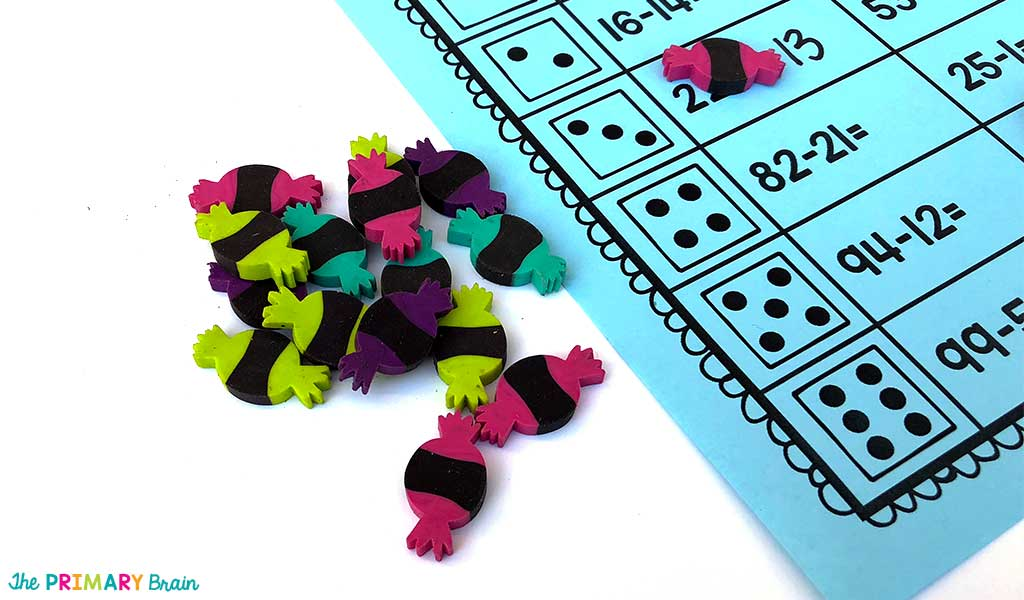This year, differentiating math instruction has been so important for my first graders. I have math levels ranging from beginning kindergarten through second grade. We have been working really hard to learn two digit addition and subtraction. One of my student’s favorite ways to practice is through Roll and Solve Math Fact equation games. For this activity, I break up my class into two groups.

### Roll and Solve Math Equations Within 20

Students who are working at a kinder math level pair up with a student who is at a beginning first grade level. These students work well together because the more advanced student can help teach the struggling student the addition and subtraction concepts. I find that my students retain the information longer when they are able to teach the strategies to a peer.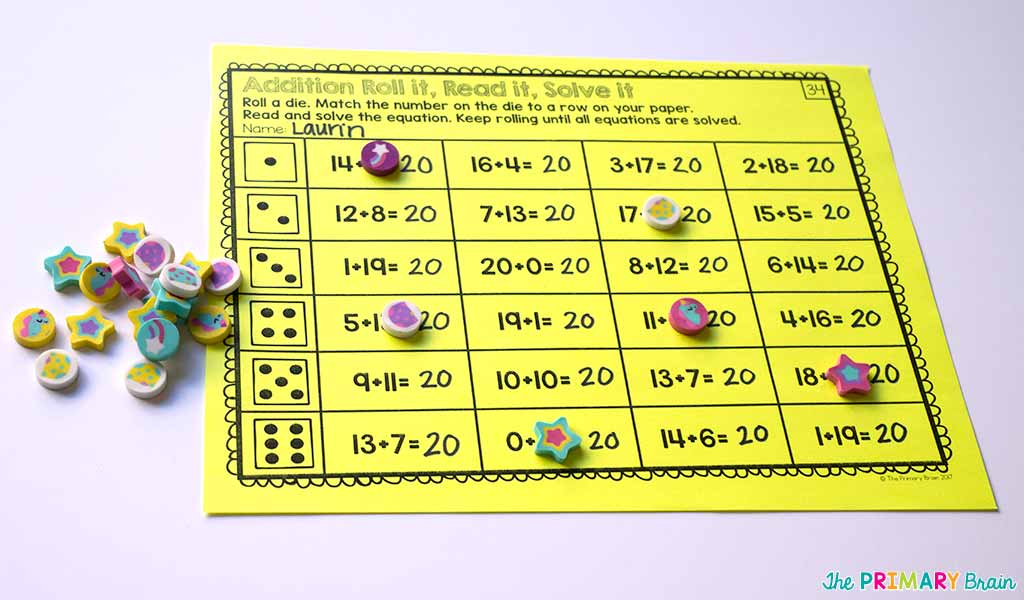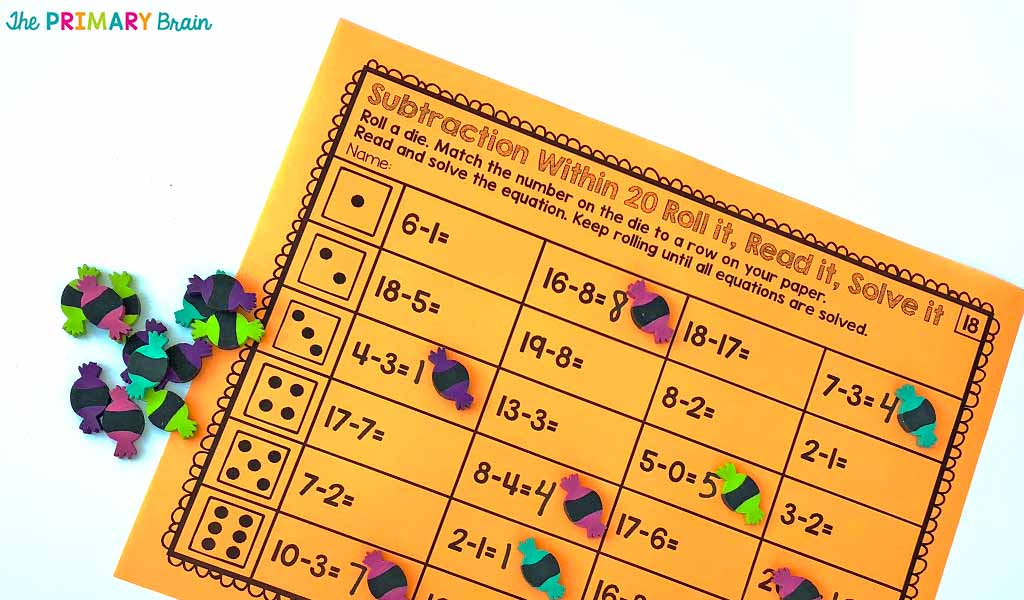Students begin the rotation by working on either an addition or subtraction exercise. To complete each game, students roll a die and answer a math fact in the cooresponding row. Each student takes a turn until all of the math equations are complete. Once students complete the entire page, they move on to the other addition or subtraction game.

### Roll and Solve Math Equations Within 100

Students who have mastered addition and subtraction of whole numbers to 20 move on to Roll and Solve within 100. This can be a challenging activity for many students since they are expected to add or subtract two double digit numbers. I always have manipulatives and charts available to assist students with solving these equations. The most helpful materials have been tens sticks and ones cubes with a tens/ones t-chart.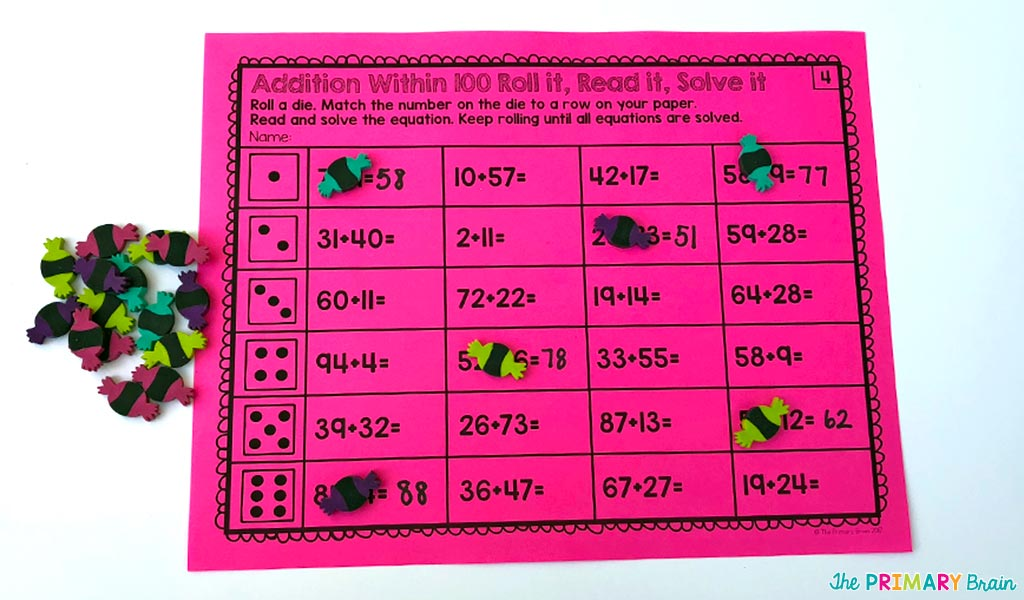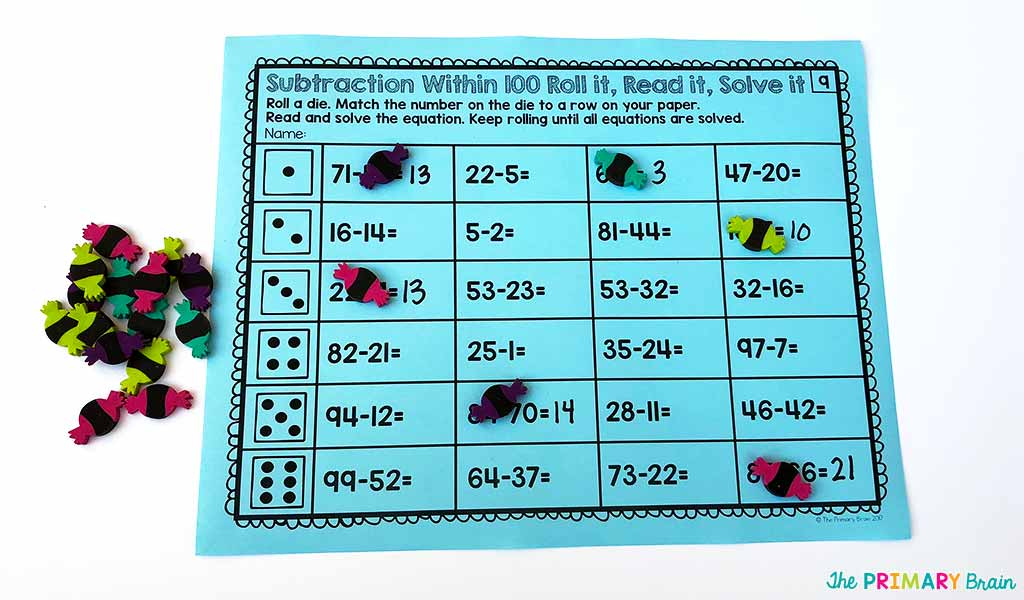Students also complete this activity by rolling a die and solving math fact equations until all math equations are answered. When students complete the first activity, then they move on to the second activity.

These activities are perfect for math centers, rainy days, or early finishers since you can laminate and use again and again. Just give students an expo marker and some fun Target erasers and you have a ready to go math activity. You can find all of these math games in my TpT store by clicking on the links below.

Roll and Solve Addition Within 20

Roll and Solve Subtraction Within 20

Roll and Solve Addition Within 100

Roll and Solve Subtraction Within 100

Save big by buying the BUNDLE! Roll and Solve Addition and Subtraction BUNDLE